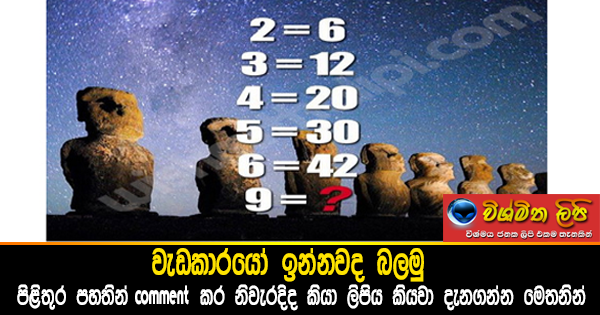1

# Can you solve this?jevldrfhda bkakjo n,uq

2*3 = 6
3*4 = 12
4*5 = 20
5*6 = 30
6*7 = 42
9*10 = 90